Area Of Cube/ Cuboid

Chapter 13 Class 9 Surface Areas and Volumes (Term 2)
Concept wise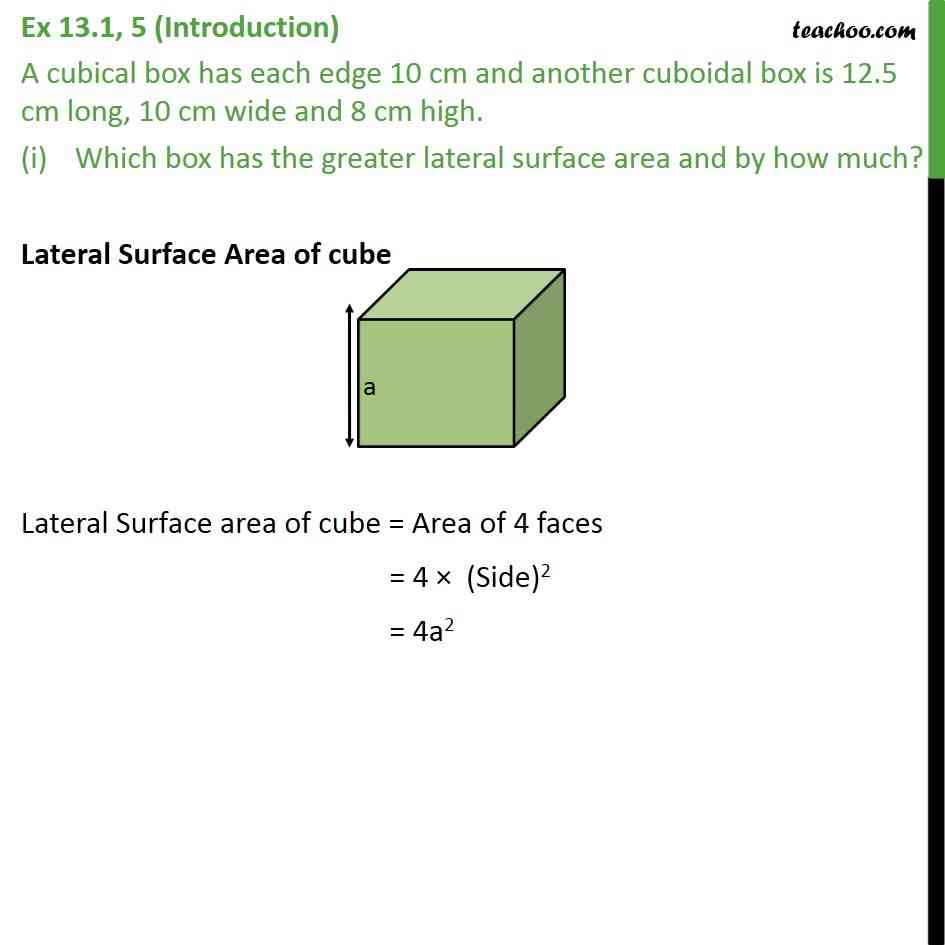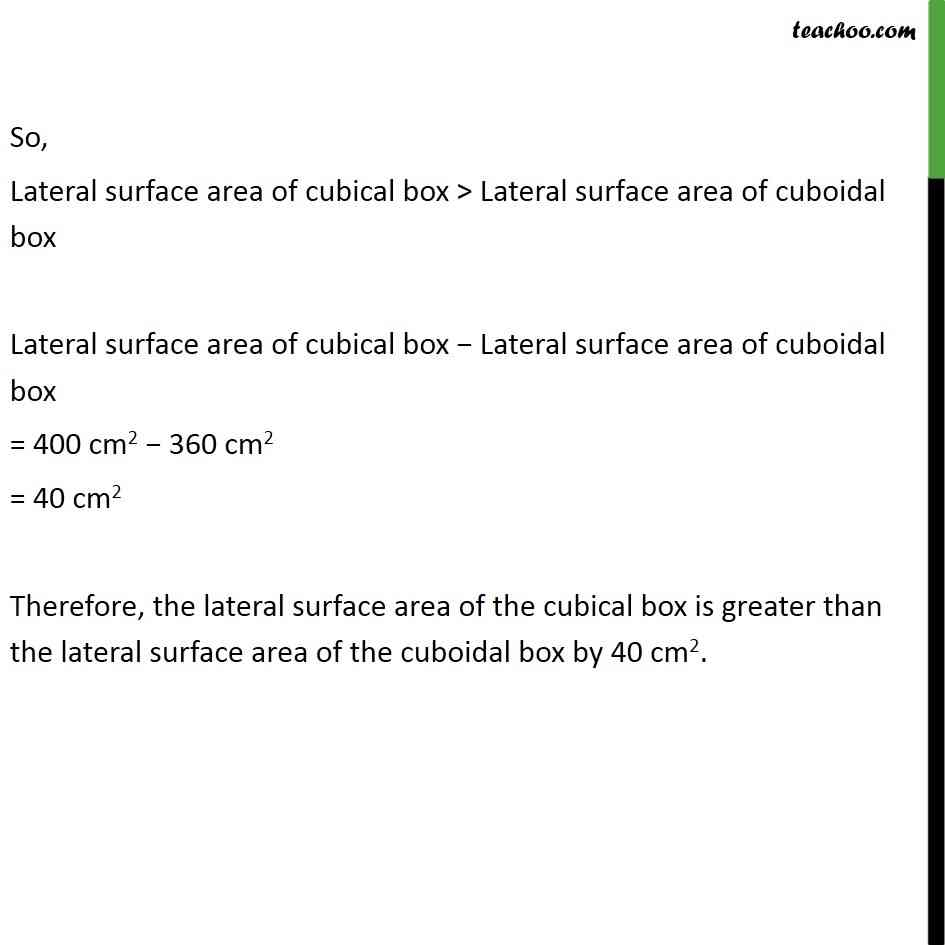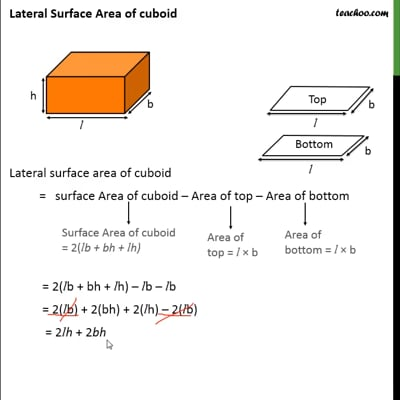This video is only available for Teachoo black users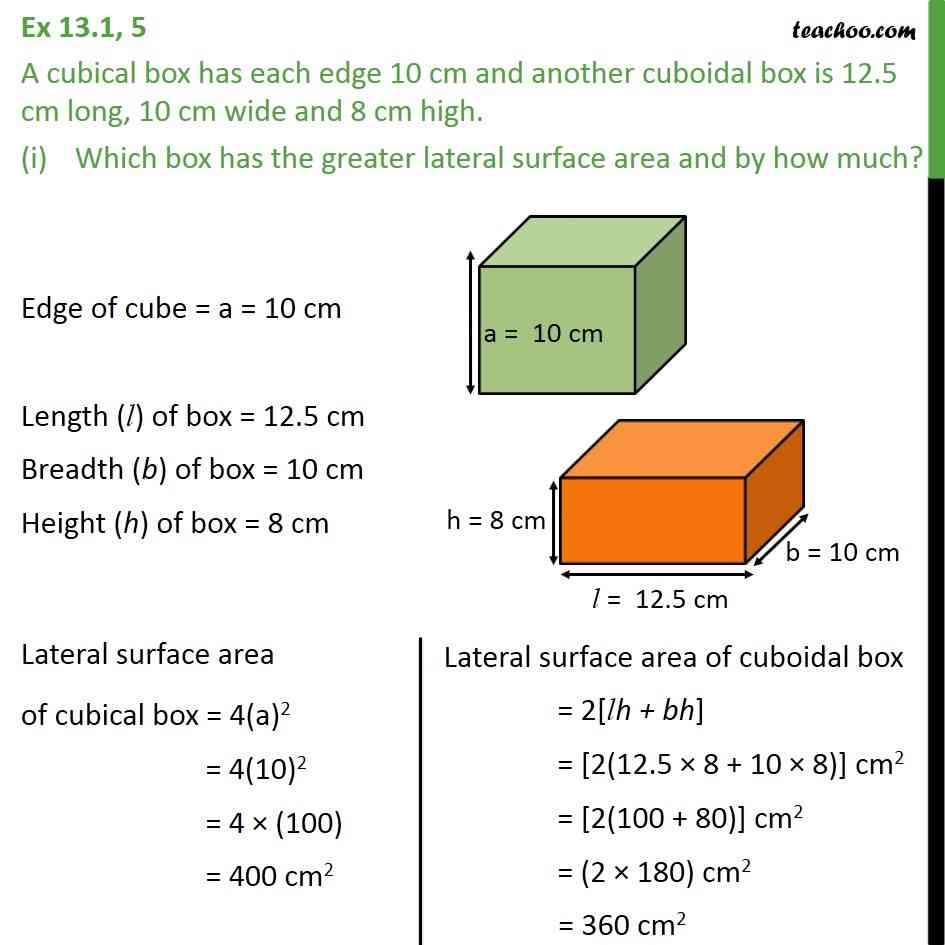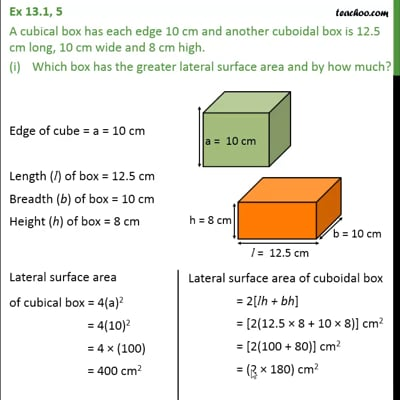This video is only available for Teachoo black users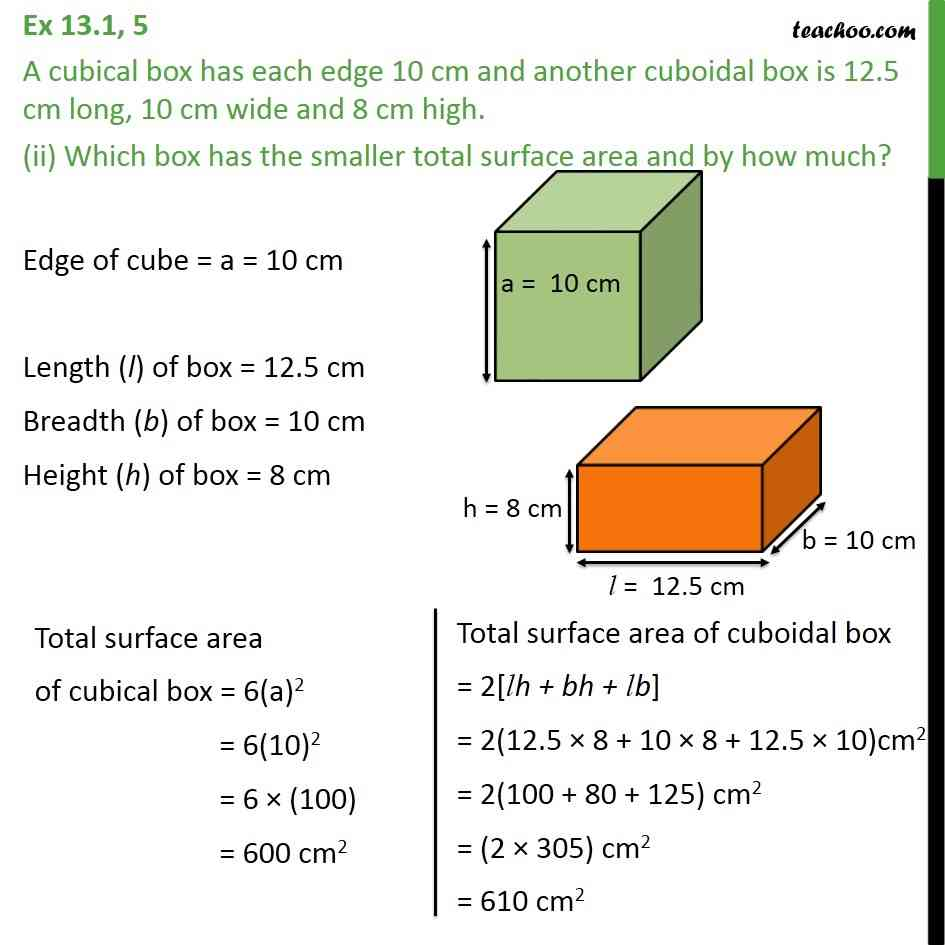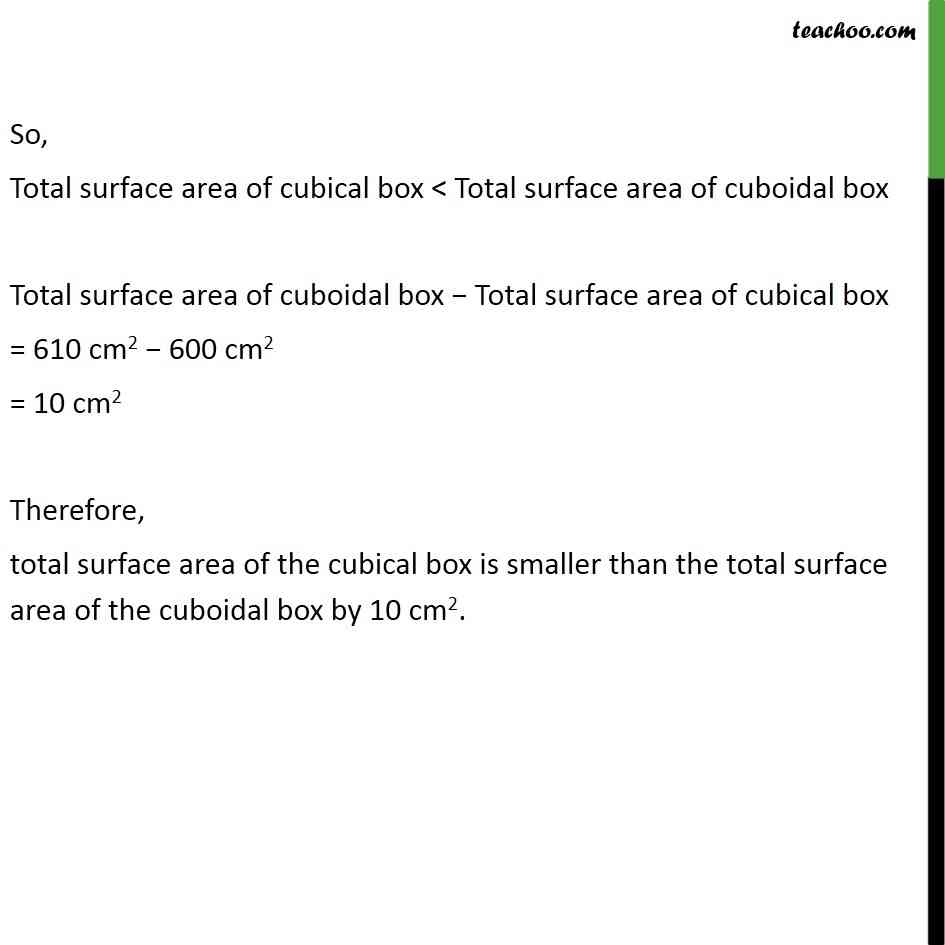In the video, calculation at the end is incorrect.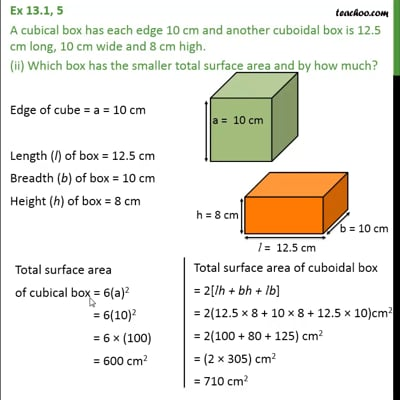This video is only available for Teachoo black users

### Transcript

Ex 13.1, 5 (Introduction) A cubical box has each edge 10 cm and another cuboidal box is 12.5 cm long, 10 cm wide and 8 cm high. Which box has the greater lateral surface area and by how much? Lateral Surface Area of cube Lateral Surface area of cube = Area of 4 faces = 4 × (Side)2 = 4a2 Lateral Surface Area of cuboid Lateral surface area of cuboid = surface Area of cuboid – Area of top – Area of bottom = 2(lb + bh + lh) – lb – lb = 2(lb) + 2(bh) + 2(lh) – 2(lb) = 2lh + 2bh Ex 13.1, 5 A cubical box has each edge 10 cm and another cuboidal box is 12.5 cm long, 10 cm wide and 8 cm high. Which box has the greater lateral surface area and by how much? Edge of cube = a = 10 cm Length (l) of box = 12.5 cm Breadth (b) of box = 10 cm Height (h) of box = 8 cm Lateral surface area of cubical box = 4(a)2 = 4(10)2 = 4 × (100) = 400 cm2 So, Lateral surface area of cubical box > Lateral surface area of cuboidal box Lateral surface area of cubical box − Lateral surface area of cuboidal box = 400 cm2 − 360 cm2 = 40 cm2 Therefore, the lateral surface area of the cubical box is greater than the lateral surface area of the cuboidal box by 40 cm2. Ex 13.1, 5 A cubical box has each edge 10 cm and another cuboidal box is 12.5 cm long, 10 cm wide and 8 cm high. (ii) Which box has the smaller total surface area and by how much? Edge of cube = a = 10 cm Length (l) of box = 12.5 cm Breadth (b) of box = 10 cm Height (h) of box = 8 cm So, Total surface area of cubical box < Total surface area of cuboidal box Total surface area of cuboidal box − Total surface area of cubical box = 710 cm2 − 600 cm2 = 110 cm2 Therefore, total surface area of the cubical box is smaller than the total surface area of the cuboidal box by 110 cm2.